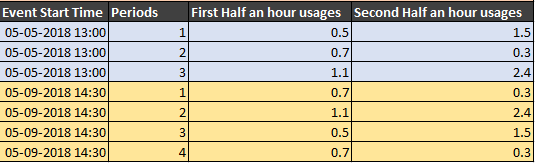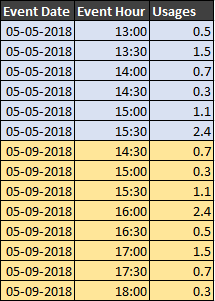# New to QlikView

Discussion board where members can get started with QlikView.

Announcements
QlikWorld, June 24-25, 2020. Free virtual event for DI and DA gurus. Register Now
cancel
Showing results for
Did you mean:
HighlightedCreator

## Need Transform Logic

Hi All,

I have a table like below INPUT format

INPUTwhat is the best way to transfer above table to get OUTPUT format

OutputPlease find the attachment for Sample Data.

Thanks

Tags (1)
1 Solution

Accepted Solutions
HighlightedMVP

## Re: Need Transform Logic

Try this

Table:

If([Event Start Time] = Previous([Event Start Time]), RangeSum(Peek('NewTime'), 1/48), 0) as NewTime,

Date(Floor([Event Start Time])) as [Event Date],

Time(Frac([Event Start Time]) + If([Event Start Time] = Previous([Event Start Time]), RangeSum(Peek('NewTime'), 1/48), 0)) as [Event Hour],

Periods,

If(IterNo() = 1, [First Half an hour usages], [Second Half an hour usages]) as Usages

FROM

(ooxml, embedded labels, table is Sheet2)

While IterNo() <= 2;

HighlightedMVP

## Re: Need Transform Logic

Try this

Table:

If([Event Start Time] = Previous([Event Start Time]), RangeSum(Peek('NewTime'), 1/48), 0) as NewTime,

Date(Floor([Event Start Time])) as [Event Date],

Time(Frac([Event Start Time]) + If([Event Start Time] = Previous([Event Start Time]), RangeSum(Peek('NewTime'), 1/48), 0)) as [Event Hour],

Periods,

If(IterNo() = 1, [First Half an hour usages], [Second Half an hour usages]) as Usages

FROM